Miscellaneous

Chapter 8 Class 11 Sequences and Series
Serial order wise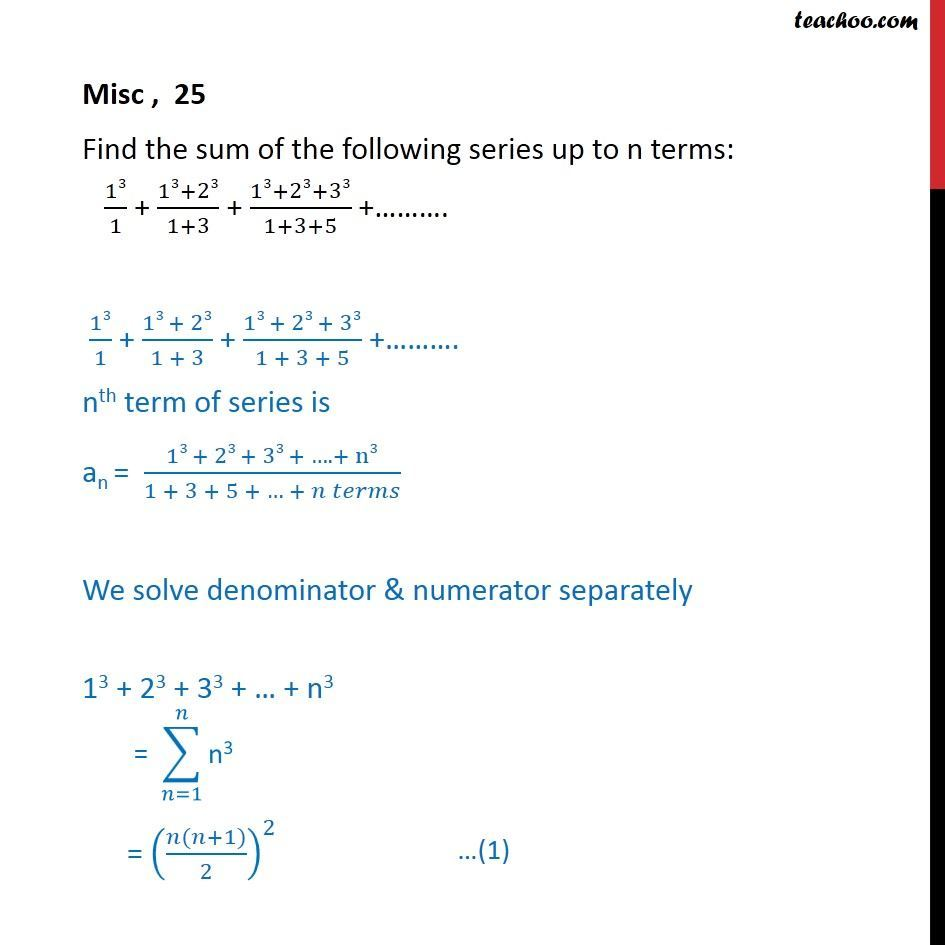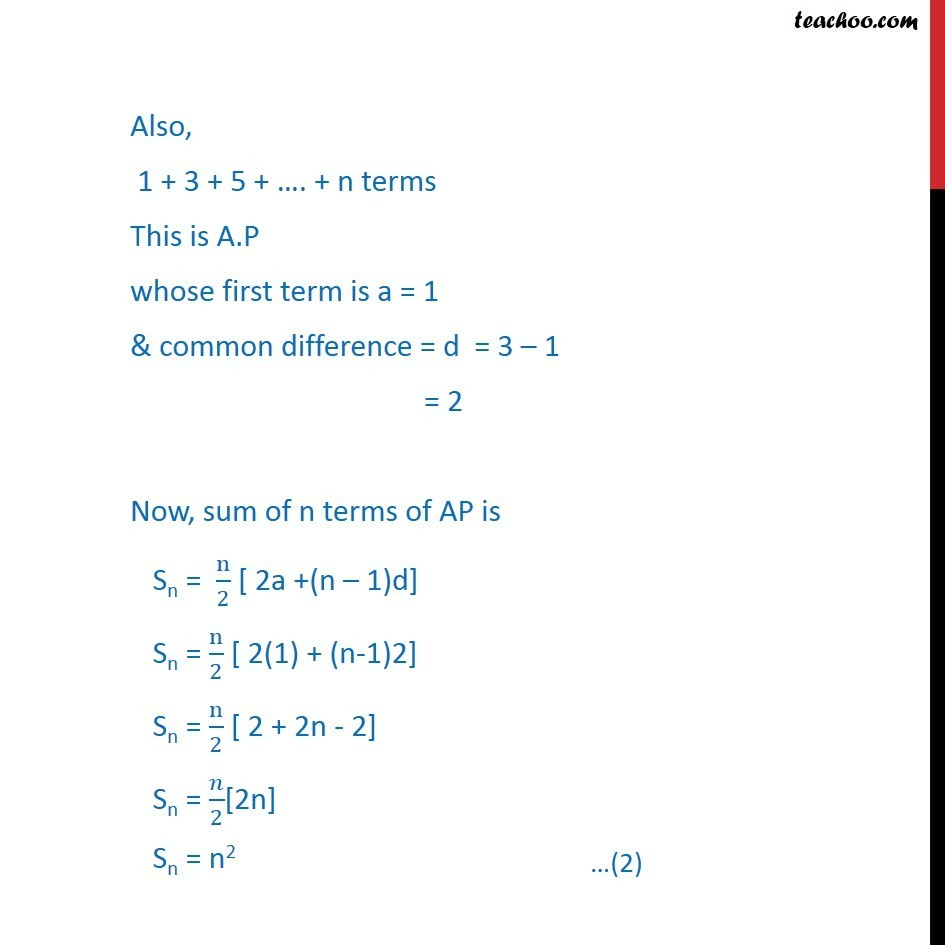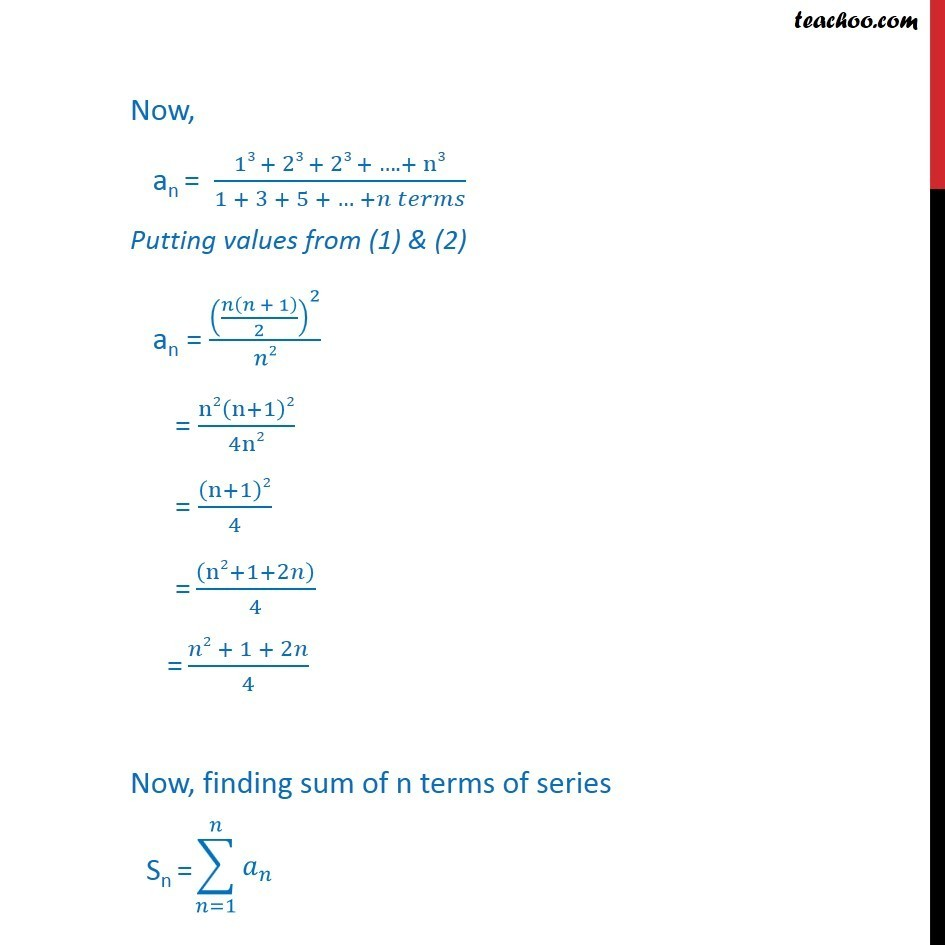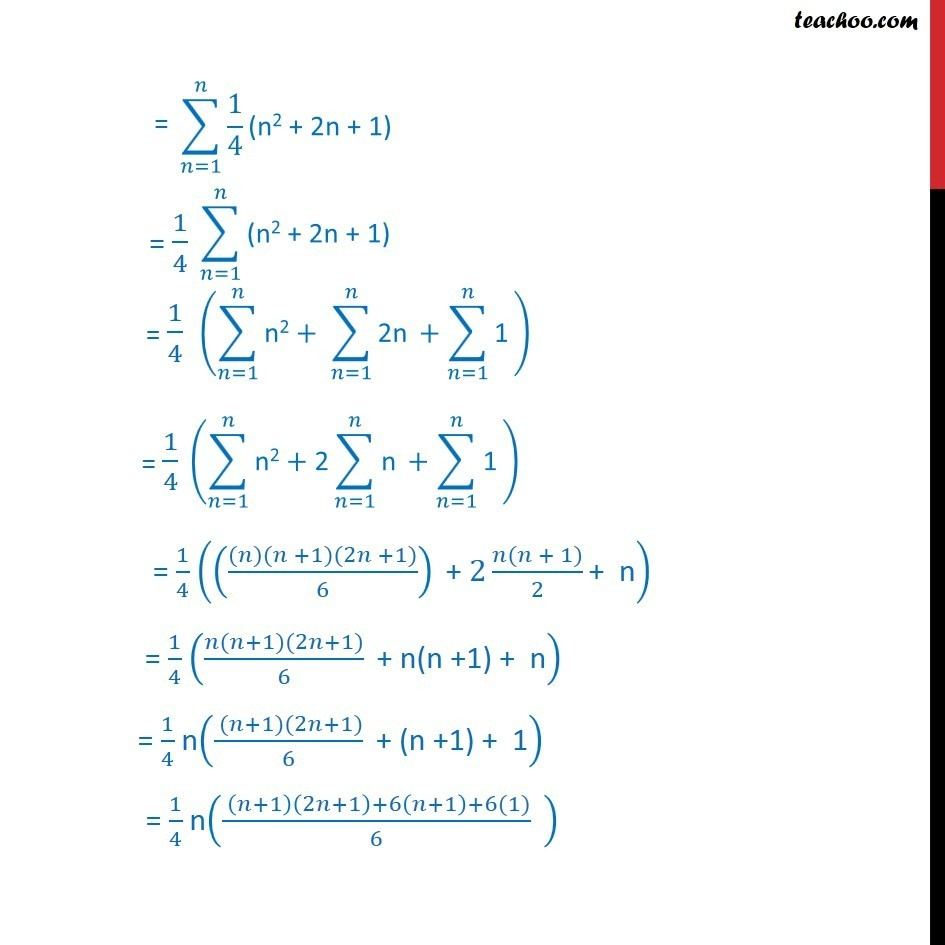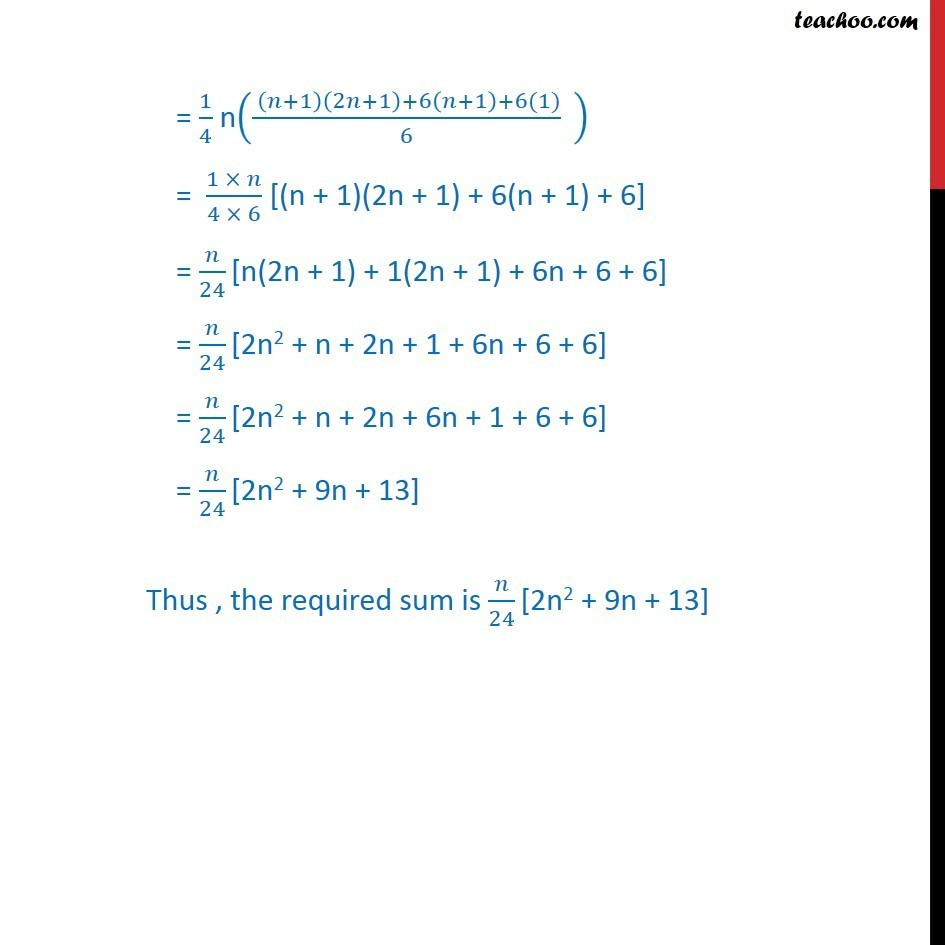Learn in your speed, with individual attention - Teachoo Maths 1-on-1 Class

### Transcript

Misc , 25 Find the sum of the following series up to n terms: 13/1 + (13+23)/(1+3) + (13+23+33)/(1+3+5) +………. 13/1 + (13 + 23)/(1 + 3) + (13 + 23 + 33)/(1 + 3 + 5) +………. nth term of series is an = (13 + 23 + 33 + …. + n3)/(1 + 3 + 5 + … + 𝑛 𝑡𝑒𝑟𝑚𝑠) We solve denominator & numerator separately 13 + 23 + 33 + … + n3 = ((𝑛(𝑛+1))/2)^2 Also, 1 + 3 + 5 + …. + n terms This is A.P whose first term is a = 1 & common difference = d = 3 – 1 = 2 Now, sum of n terms of AP is Sn = n/2 [ 2a +(n – 1)d] Sn = n/2 [ 2(1) + (n-1)2] Sn = n/2 [ 2 + 2n - 2] Sn = 𝑛/2[2n] Sn = n2 Now, an = (13 + 23 + 23 + …. + n3)/(1 + 3 + 5 + … +𝑛 𝑡𝑒𝑟𝑚𝑠) Putting values from (1) & (2) an = (𝑛(𝑛 + 1)/2)^2/𝑛2 = n2(n+1)2/4n2 = (n+1)2/4 = ((n2+1+2𝑛))/4 = (𝑛2 + 1 + 2𝑛)/4 Now, finding sum of n terms of series = 1/4 ((((𝑛)(𝑛 +1)(2𝑛 +1))/6)" + " 2 (𝑛(𝑛 + 1))/2 "+ n" ) = 1/4 ((𝑛(𝑛+1)(2𝑛+1))/6 " + n(n +1) + n" ) = 1/4 n(( (𝑛+1)(2𝑛+1))/6 " + (n +1) + 1" ) = 1/4 n(( (𝑛+1)(2𝑛+1)+6(𝑛+1)+6(1))/6 " " ) = 1/4 n(( (𝑛+1)(2𝑛+1)+6(𝑛+1)+6(1))/6 " " ) = (1 × 𝑛)/(4 × 6) [(n + 1)(2n + 1) + 6(n + 1) + 6] = 𝑛/24 [n(2n + 1) + 1(2n + 1) + 6n + 6 + 6] = 𝑛/24 [2n2 + n + 2n + 1 + 6n + 6 + 6] = 𝑛/24 [2n2 + n + 2n + 6n + 1 + 6 + 6] = 𝑛/24 [2n2 + 9n + 13] Thus , the required sum is 𝑛/24 [2n2 + 9n + 13]